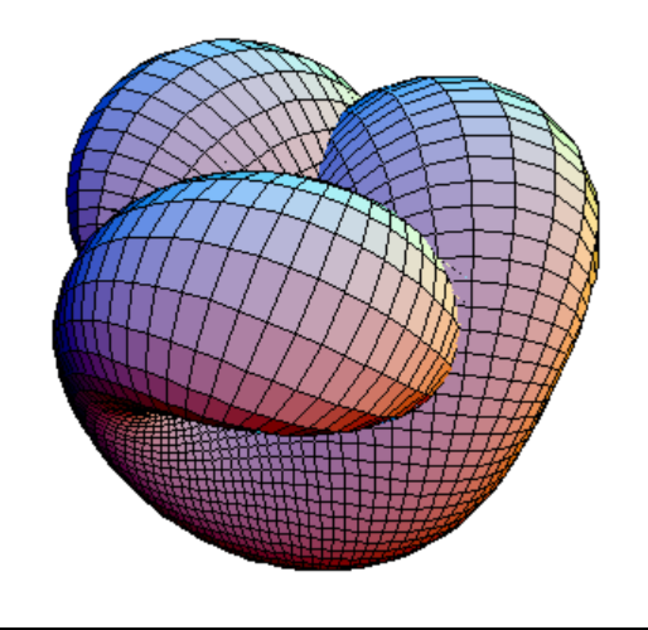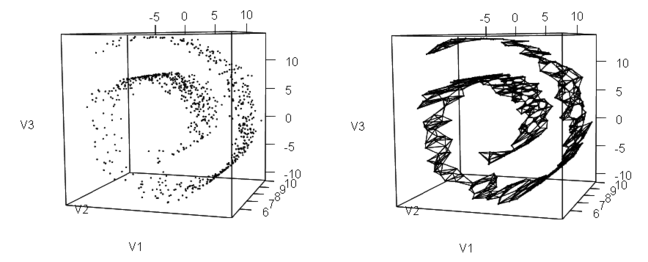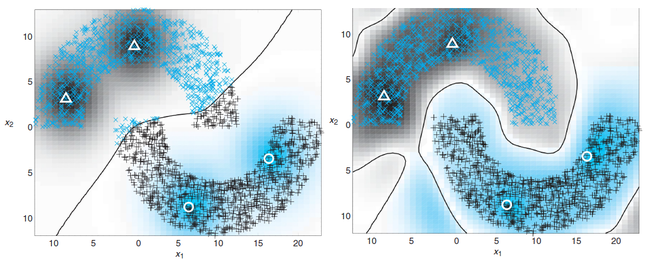## Want to keep learning?

This content is taken from the The Open University & Persontyle's online course, Advanced Machine Learning. Join the course to learn more.
4.21

# Laplacian Regularized Least Squares

## Semi-Supervised Learning Recap

Semi-supervised learning deals with cases similar to supervised learning except that we do not have labels (values for the target variable) for all our training data. Typically, the number of unlabelled cases is significantly higher than the number of laballed cases. Being able to work with such data can be very useful as many real life problems are such that unlabelled data is easily available but labelled data is difficult and expensive to obtain. In an example from our work, we have attempted to detect ISIS posts among social media data. We obtained millions of social media posts, but had to manually label them as being ISIS or not. Being able to only label a small number and then use all available posts through semi-supervised learning is a lot quicker and cheaper than paying someone to label sufficiently many to be able to use a supervised learning algorithm.

## Laplacian Regularized Least Squares

You may need torefresh your understanding of kernel regression and the representer theorem. If so, re-read the Basics & Kernel Regression step of week two.

The semi-supervised learning algorithm we will look at here is a kernel based approach called Laplacian regularized least squares. It takes as a basis an L2 regularized kernel regression model. As previously noted, when performing L2 regularization for a model of some form, $$f$$, we seek to solve the optimization problem:

$\hat{f}(x)=min_{f} \sum_{i=1}^n(y_i-f(x_i))^2+\lambda \|f\|^2$

We also noted that when performing kernel regression with kernel $$k$$, it is an important result of regularization theory that the minimizer of the above equation will be of the form:

$\hat{f}(x)=\sum_{i=1}^n \alpha_i k(x,x_i)$

With $$\alpha$$ calculated as described in the Basics & Kernel Regression step.

The semi-supervised component of the algorithm takes the form of restricting the above equations to the labelled data cases, and additionally penalizing dissimilar estimates of near neighbors in the feature space. To do this, we will add an additional term to the optimization problem:

$\hat{f}(x)=min_{f} \sum_{i=1}^l(y_i-f(x_i))^2+\lambda_R \|f\|^2+\lambda_S M(f)$

Which is equivalent to:

$\hat{f}(x)=\sum_{i=1}^l \alpha_i k(x,x_i)+\lambda_S M(f)$

Note that we have used the superscript $$l$$ rather than $$n$$ in the summations of both these equations to indicate that we are summing over the labelled cases only. We will reserve n for the total number of training cases, labelled or unlabelled.

What we want is to provide some principled specification of M such that it too involves only closed form operations on the feature vectors or kernel operations thereof. In doing so, we will make two, generally plausible, assumptions, known as the manifold and cluster assumptions.

#### The manifold assumption:

The marginal distribution of the feature vectors in the input space is supported on a low-dimensional manifold.

Manifolds are topological spaces that locally resemble Euclidean space, such that, say, a warped two dimensional plane in three dimensional space is a two dimensional manifold. An example of such a manifold is the surface of the Earth, and the real projective plane, given below, is another (albiet quite a complicated one!). This image is from wikipedia, where you can also find more information and visual examples.#### The cluster assumption:

The marginal distribution according to which the feature vectors are generated is such that if certain points are located near each other then they are likely to have the same or similar target values. This, of course, is something that is almost always assumed in machine Learning, but here we make this assumption regarding the locations of the feature vectors on the manifold.

## Representing the feature manifold

We will seek to provide a representation of near feature vectors on the generating manifold by trying finding an undirected graph representation of the data-manifold in the feature space.

To do this we generate a proximity graph of our data, almost as described in the Graph Clustering section of the Clustering: Introduction step in week three. Re-read this section if required. Although any dissimilarity measure can be used to generate the proximity matrix, it is normally done using Euclidean distance.

We then specify an adjacency criterion (dissimilarity threshold), such that two data vectors will be considered connected in our undirected graph, $$G$$, if and only if they are within some distance, $$\epsilon$$, from each other. We will produce an undirected graph from data similar to that shown in the image below (on the ‘Swiss roll’ dataset).We will then give the edges of this graph a weight based not on the Euclidean distances of the feature vectors but instead using our chosen kernel such that the weight, $$w_{i,j}$$, of the edge between two connected feature vectors, $$x_i$$ and $$x_j$$, will be $$k(x_i,x_j)$$. This will result in a weight matrix, $$W$$ such that only feature vectors close to each other in the input space will have non-zero values, and that such non-zero values will equal the kernel function of the two close feature vectors.

We also create the n by n diagonal matrix $$T$$, where the element $$t_{i,i}=\sum_{j=1}^n w_{i,j}$$, which is the (weighted) degree of node i. We are now able to calculate what is called the Laplacian of our graph, $$L=T-W$$.

With this we are able to define $$M$$ as:

$M(f)=F^TLF$

Where $$F=[f(x_1),f(x_2),...,f(x_n)]$$.

Using this as the third term in our optimization problem will penalize model functions for giving dissimilar estimates of the target variables to input vectors that are close to each other on a discovered data-manifold in the input space. Don’t worry if you have a hard time ‘intuitively understanding’ the process explained - it takes a while to get used to this - so long as you understand what it is we have done.

## The Generalized Representer Theorem

Given the above, we are able to rewrite our semi-supervised optimization problem as:

$\hat{f}(x)=min_{f} \sum_{i=1}^l(y_i-f(x_i))^2+\lambda_R \|f\|^2+\lambda_S F^TLF$

The generalized representer theorem, which we will not prove, states that, for a given kernel k and chosen values of the regularization parameters $$\lambda_R$$ and $$\lambda_S$$, the optimal solution to this optimization problem is of the form:

$\hat{f}(x)=\sum_{i=1}^l\alpha_i^* k(x,x_i)$

The optimal $$\alpha^*$$ coefficients can be calculated by the following closed-form matrix expression:

$\alpha^* = (JK + \lambda_R I + \lambda_S LK)^{-1}J^Td$

Where d is a l by 1 vector given the known target variable values of the labelled training data, $$d = [y_1, y_2,..., y_l]$$, J is a diagonal n by n matrix whose diagonal consists of l ones followed by n-l zeros, K is the l by l gram matrix, and L is the graph Laplacian matrix.

## Example

Since we will not be able to quickly create an R script to show an application of the LRLS algorithm, we borrow an example, and an associated image, from Simon Haykin’s Neural Networks and Learning Machines (3rd Edition, Pearson Education, 2011), which can also be read to provide more detail on the ideas given above.Here we have a case where we are performing classification using kernel regression (the binary classes are treated as 0 / 1 valued and regression is performed) on the famous ‘half moon’ dataset. We have many training cases, but only four labelled cases, indicated in the images. We see the dramatic improvement in performance when performing semi-supervised learning with LRLS over just performing supervised learning on the handful of labelled training data cases.